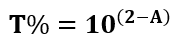# Absorbance to Transmittance Conversion Calculator

This is an online calculator that calculates the Light Transmittance of any medium. Just enter the Absorbance or Optical Density value to get the corresponding Light Transmittance in Percentage.

### Result

• Light Transmittance %
##### Image for Absorbance to Transmittance Conversion CalculatorTransmittance of any medium is the amount of light that passes through the medium and comes out of the other side. It is defined as the ratio of the intensity of the transmitted light to the incident light.

Let Io be the intensity of the incident light and I be the intensity of the transmitted light. Then transmittance T of the medium is mathematically given as,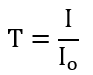Absorbance of any medium is the amount of light that the medium absorbs when a light passes through it and thereby reducing the intensity of the incident light. It is defined as the logarithm of the ratio of incident to transmitted light intensity through a medium.

Let Io be the intensity of the incident light and I be the intensity of the transmitted light. Then absorbance A of the medium is mathematically given as,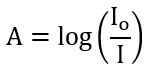Rewriting the equation, we get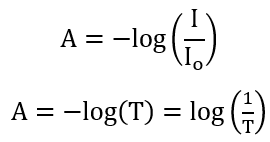Transmittance value in (%) is given as T x 100%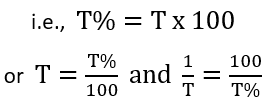So,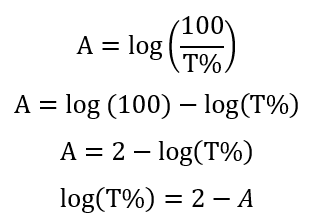From the above equation, we can write the transmittance of any medium in (%) as,